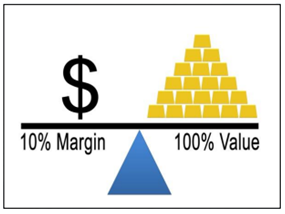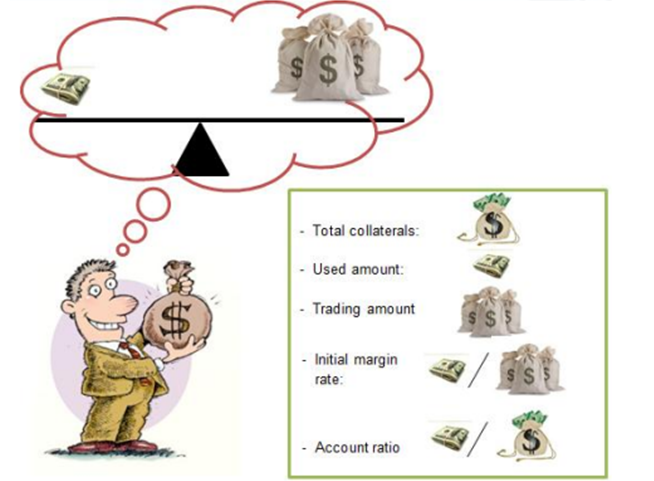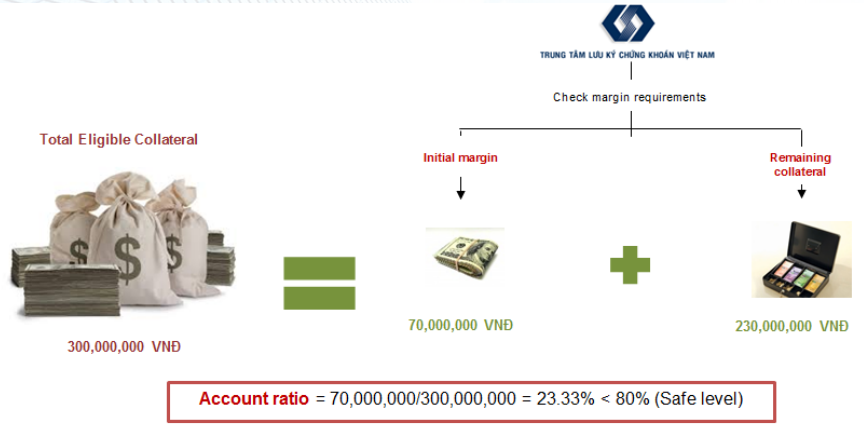# Knowledge

## Đấu giá mới nhất

Knowledge

During a trading day, profits/losses will fluactuate with real-time market price (mark – to – market)

At the end of a trading day, all profits/losses of closed and open positions are realized and paid to investors.

For open positions, VSD will calculate profits/losses as followings:

Positions opened in the trading day:
Profits/losses = (Daily settlement price – Executed price) * Multiplier * Number of contracts
Positions opened in previous days:
Profits/losses = (Daily settlment price – Previous-day settlment price) * Multiplier * Number of contracts

Leverage refers to using little amount of outlay to control large positions in derivatives trading.

For example, investor A decides to BUY 10 index futures contracts at price 700 and maturity date December 2017:

 Contract code Quantity Executed price Multiplier VN30F1712 10 (LONG) 700 100,000

 If initial margin rate is 10%: Trading amount = 700 * 10 * 100,000 = 700,000,000 VND Initial margin = 10% * 700,000,000 = 70,000,000 VND Leverage effect = 700,000,000 / 70,000,000 = 10 times   If initial margin rate is 15%: Trading amount = 700 * 10 * 100,000 = 700,000,000 VND Initial margin = 15% * 700,000,000 = 105,000,000 VND Leverage effect = 700,000,000 / 105,000,000 = 6.67 timesInitial margin refers to the minimum collateral values that investors must have to trade a specific derivatives contract. Initial margin rate can be used to calculate leverage effect of the contract. Account ratio is the proportion of collaterals used for derivatives trading. Investors can use this figure to calculate the remaining values of collateral assets for next transactions.Account ratio = Margin Requirements / Total Eligible Collateral amount

In which:

Margin requirements = IM + VM + SM + DM

IM = initial margin
VM = variation margin (net loss = unrealized profits/losses + realized profits/losses)
SM = spread margin (currently, not applicable)
DM = delivery margin (initial margin applied for physical delivery)

Total Eligible Collateral amount = Cash + Eligible securities

Account ratio will vary real-time with market prices of derivatives contracts

For example:

Step 1. An investor deposits 240 million VND in cash and 100 million VND in stock as collaterals for derivatives trading.

To ensure the minimum proportion of cash collateral is 80%, Total Eligible Collateral will be: 240 million + min (100 million; 25% * 240 million) = 300 million VNDStep 2. The investor expects the market will go “up”, thus he decides to BUY index futures contracts matured at December 2017

 Contract code Quantity Executed price Multiplier IM rate VN30F1712 10 (LONG) 700 100,000 10%Step 3. VSD checks Initial margin requirment of the transaction

 Contract code Initial margin Margin requirements Total eligible collateral VN30F1712 10 * 700 * 100,000 * 10% = 70,000,000 VND 70,000,000 VND (Initially, VM = 0) 300,000,000 VND

Step 4. VSD checks Account ratio of the trading accountStocks Futures First trading day The first day listed in stock exchange - The first day listed in stock exchange - The number of listed contracts does not vary: new contracts will be automatically listed after a contract is matured Listing volume Limited by issuing amounts of stocks Unlimited Number of contract codes Each stock code is corresponding to an individual stock/bond/fund certificate Each contract has different codes corresponding to contract months Short-sell Not allow to short sell Allow to short sell Final trading day The day before that stocks are unlisted The final day that futures contracts have value Payment period T+3 Daily settlement Payment method Physical delivery Cash settlement and physical delivery Payment mechanism Exchange stocks and cash Central Counterparties (CCP) Margin Not compulsory (a facility that brokerage firms provide to customers) Compulsory to ensure payment ability Subjects of margin Buyers Buyers and sellers